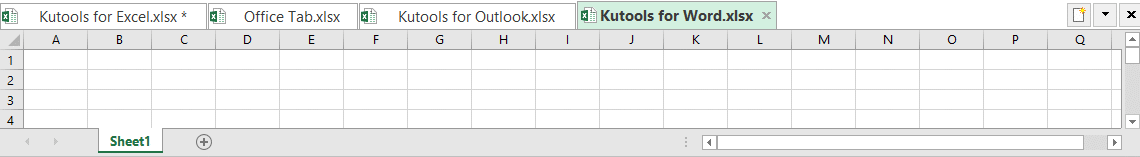## EXCEL COUNTIF function – Count cells that are not blank, greater/less than, or contain a specific value

When working on an Excel worksheet, to count the number of cells, such as to count blank or non-blank cells, cells greater than or less than a given value, or cells that contain a specific text may be some common tasks for most of us. To deal with these calculations, the COUNTIF function in Excel may do you a favor.

Syntax of COUNTIF function in Excel

COUNTIF function for blank or non-blank cells

COUNTIF function for cells less than, greater than, or equal to a specific value

COUNTIF function for cells contain a specific value

#### Syntax of COUNTIF function in Excel

As one of the statistical functions in Excel, COUNTIF counts cells that meet given criteria in a specified range. The syntax of the COUNTIF function is:

=COUNTIF(range, criteria)

The syntax contains two arguments – range and criteria:

• range: refers to the range of cells you want to count.
• criteria: refers to the condition you want to use to count cells. For example, you can use “>=80” as a criterion to look for the cells with values that are greater than or equal to 80 in the range you selected.

To apply the function in Excel, you should type =COUNTIF(range, criteria) in a destination cell. For example, =COUNTIF(A1:B8,">=80").

The COUNTIF function returns a numeric value – the number of the cells you wanted to count.

Now that we have a clearer understanding of the COUNTIF function, let’s move forward to some real examples.

#### COUNTIF function for blank or non-blank cells

For example, I have a list of cells that contains different data types, such as text, Boolean values (TRUE and FALSE), numbers, dates, and errors. Is there a universal COUNTIF formuala to count only blank or non-blank cells no matter what data types exist in your specified range?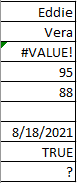##### Count blank cells with COUNTIF function

Now, let me introduce the COUNTIF formula to you. With the formula, no matter how many data types exist in the range, it will tell you the exact and correct number of the empty cells:

=COUNTIF(range,"")
Note: There is no text in quotes, which means the cells you want to count are blank.

To use the COUNTIF function to count empty cells, type the formula =COUNTIF(A1:A9,"") in a destination cell, then press ENTER: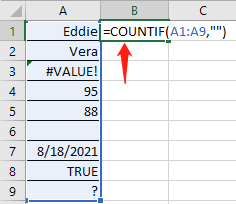>>>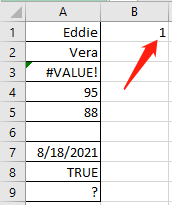In excel, there is also a function called COUNTBLANK, which returns the number of empty cells in a specified range. The syntax of it is =COUNTBLANK(range). For the example above, you can use the formula: =COUNTBLANK(A1:A9).

##### Count non-blank cells with COUNTIF function

To count the cells that are not empty, here, let me show you the formula:

=COUNTIF(range,"<>")
Note: <> in Excel means not equal to. So, the formula above counts all the cells that are not equal to blank, or we can say, are not blank.

To use the COUNTIF function to count the cells that are not empty, type the formula =COUNTIF(A1:A9,"<>") in a destination cell, then press ENTER: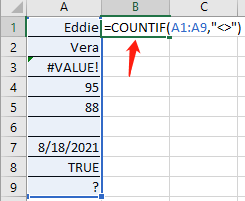>>>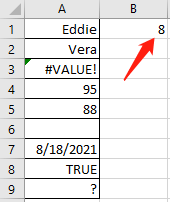In excel, there is also a function called COUNTA, which returns the number of cells containing any values in a specified range. The syntax of it is =COUNTA(range). For the example above, you can use the formula: =COUNTA(A1:A9).

However, if you only want to count cells with only text and exclude Boolean values (TRUE and FALSE), numbers, dates, or errors, please use the formula below:

=COUNTIF(A1:A9,"*")
Note: A single asterisk (*) matches only the value in a text form.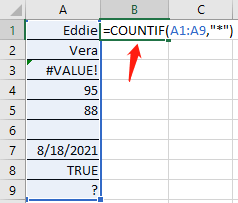>>>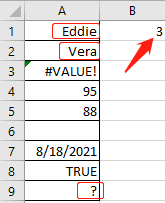##### Count non-blank cells with two clicks

If you ha installed in your excel, with its Select Nonblank Cells feature, you can select all nonblank cells at once, and get the number as you need.

1. You can go to the Kutools tab in Excel, find Select, and click Select Nonblank Cells on the drop-down list. See screenshot: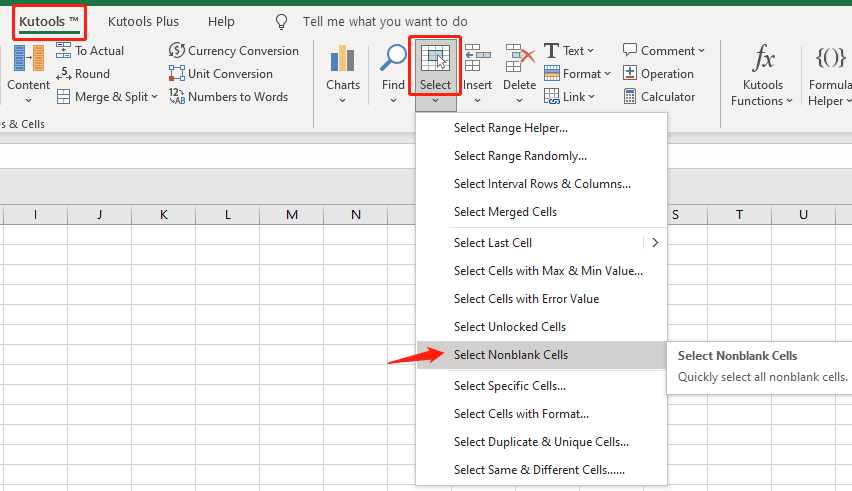2. All nonblank cells will be selected, and a prompt box will pop out to tell you the number of nonblank cells as below screenshot shown: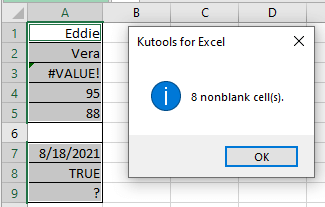#### COUNTIF function for cells less than, greater than, or equal to a specific value

Here we have listed two main methods for you to count cells with values that are less than, greater than, equal to, or not equal to a specific value in Excel.

##### COUNTIF less than, greater than, equal to, or not equal to with formulas

To use a formula to count cells with values that are less than, greater than, equal to, or not equal to a specific value, you should make good use of Comparison Operators (<, >, =, ).

Note: The method can also be used to count cells with dates that are before (less than <), after (greater than >) or equal to (=) the date you specify.

Now, let’s take a look at some real examples according to the table below: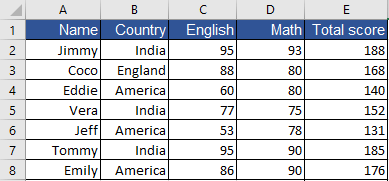To count the number of students whose total score is over (>) 140, use the formula:=COUNTIF(E2:E8,">140") >>> The result is 5 To count the number of students whose math score is lower than (<) the score of Coco (the value in the cell D3), use the formula:=COUNTIF(D2:D8,"<"&D3) >>> The result is 2√ Note: To use a cell reference in COUNTIF function with comparison operators, you will have to put the operators in quotes, and add an ampersand (&) before the cell reference. To count the number of students whose English score is over or equals to (≥) the score of Eddie (the value in the cell C4), use the formula:=COUNTIF(C2:C8,">="&C4)-1 >>> The result is 5√ Note: The reason to subtract 1 is that the criteria C2:C8,">="&C4 ask COUNTIF to count all the matching cells including the one with Eddie’s English score, so we need to subtract it from the formula. If you need to count Eddie in, delete “-1” from the formula. To count the number of students who are from (=) India, use the formula:=COUNTIF(B2:B8,"India") OR =COUNTIF(B2:B8,B2) >>> The result is 3√ Note: The value in the cell reference B2 is India, so “India” and B2 are both ok working as criteria here. In the COUNTIF function, the criteria are not case-sensitive. So, the string “India” and “INDIA” will make no difference to the result of the formula. To count the number of students who are not from (≠) India, use the formula:=COUNTIF(B2:B8,"<>India") OR =COUNTIF(B2:B8,"<>"&B2) >>> The result is 4

The COUNTIF function only works when there is one condition, for the situation of more conditions, you should use the COUNTIFS function. For example, to count the number of students whose English score is between 60 and 90 (>60, <90), use the formula: =COUNTIFS(B2:B8,">60",B2:B8,"<90").
Click to know more about the COUNTIFS function…

##### COUNTIF less than, greater than, equal to, or not equal to with few clicks

To count cells with values that are less than, greater than, equal to, or not equal to a specific value in a more convenient way, you can apply the Select Specific Cells feature o

After installing Kutools for Excel, please do with the following steps:

1. Please find the Kutools tab, then go to Select, and click Select Specific Cells on the drop-down list.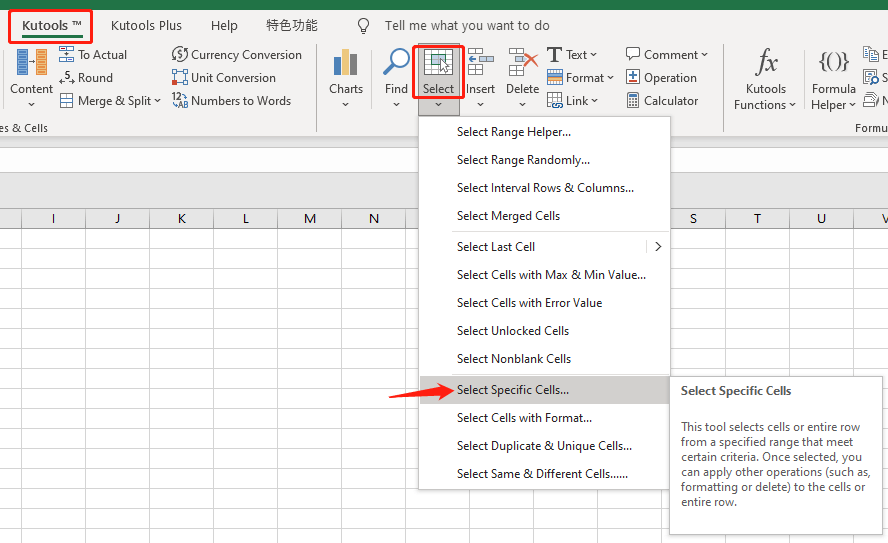2. Now you will see a pop-up window, please do as follows: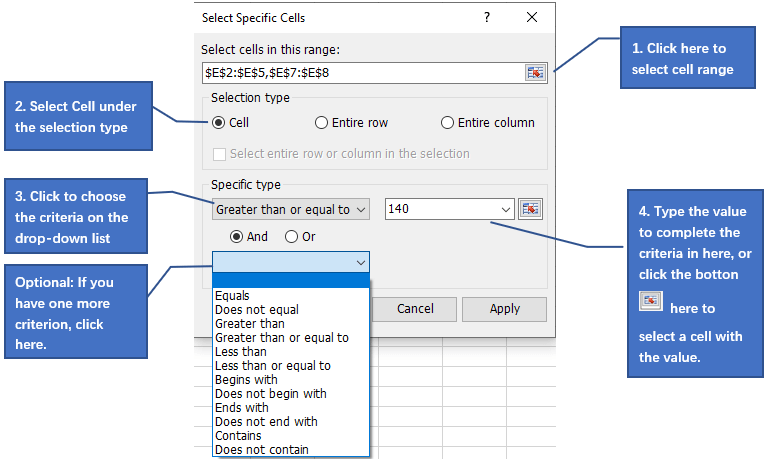Below is an example to count the number of students whose math score is lower than (<) the score of Coco with Kutools.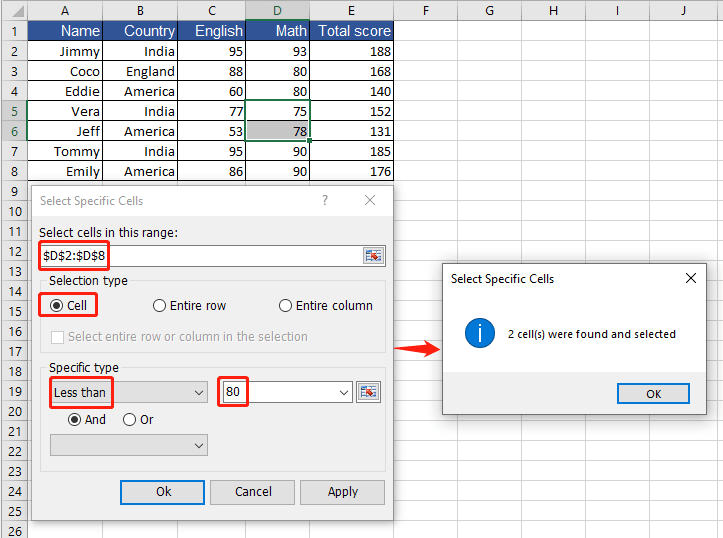Note: The program will return the count result and select the cell(s) that meet(s) your criteria. You can copy them if you need.

#### COUNTIF function for cells contain a specific value

To use COUNTIF to count the cells that contain a specific value (for example the cells with a letter Y), or even to count the cells with a specific value at a specified position (for example the cells begin with a letter Y), we have to know about the wildcard characters.

There are three wildcard characters - asterisk (*), question mark (?), and tilde (~) available in Excel for the COUNTIF function:

Asterisk (*) - Matches any number of characters. For example, *ice could mean nice, service, ice, @#\$ice, etc.
Question mark (?) - Matches any one character. For example, mo?? could mean more, moon, mo&%, moaa, etc.
Tilde (~) - Matches the actual wildcard character. For example, ~* means a literal asterisk mark, ~~ means a literal tilde.

Note: Boolean values (TRUE and FALSE), numbers, dates, and errors are not counted as characters. So if there are above elements in the cell range you selected, you will have to convert them to text.

##### Count cells containing specific word(s) or character(s) with COUNTIF function

Since we already know about the wildcard characters, it’s time for us to learn how to use a COUNTIF formula to count the cells that contain a specific character(s) or word(s). Let’s see the examples below: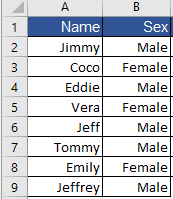To count the number of boys in the class, use the formula:=COUNTIF(B2:B9,"MALE") >>> The result is 5 To count the number of names that contains the characters “jeff” (the value in the cell A6), use the formula:=COUNTIF(A2:A9,"*jeff*") OR =COUNTIF(A2:A9,"*"&A6&"*") >>> The result is 2 To count the number of names that contain the letter “e”, use the formula:=COUNTIF(A2:A9,"*e*") >>> The result is 5 To count the number of names that don’t contain the letter “e”, use the formula:=COUNTIF(A2:A9,"<>*e*") >>> The result is 3 To count the number of names that begin with the letter “e”, use the formula:=COUNTIF(A2:A9,"e*") >>> The result is 2 To count the number of names that end with the letter “e”, use the formula:=COUNTIF(A2:A9,"*e") >>> The result is 1 To count the number of names with the letter “m” as the third character, use the formula:=COUNTIF(A2:A9,"??m*") >>> The result is 2

##### Count cells containing specific word(s) or character(s) with few clicks

1. Go to the Kutools tab, find Select, and click Select Specific Cells on the drop-down list.2. In the Select Specific Cells dialog box, select the cell range you want to count, and then click Cell under the Selection type, choose one condition in the drop-down list of the Specific type according to your needs.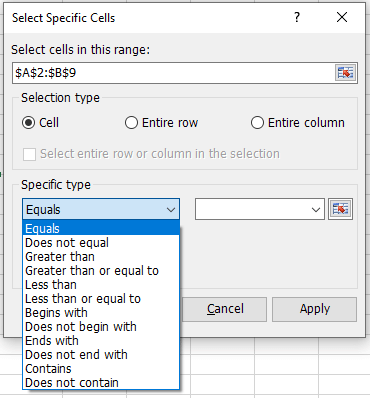Here, I will give you two examples of using Kutools to count the cells that contain the specific word(s) or character(s).

• To count the number of boys in the class, click Equals on the drop-down list, and type Male in the right input box, or you can select a cell with the value “Male” in the worksheet by clicking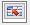button.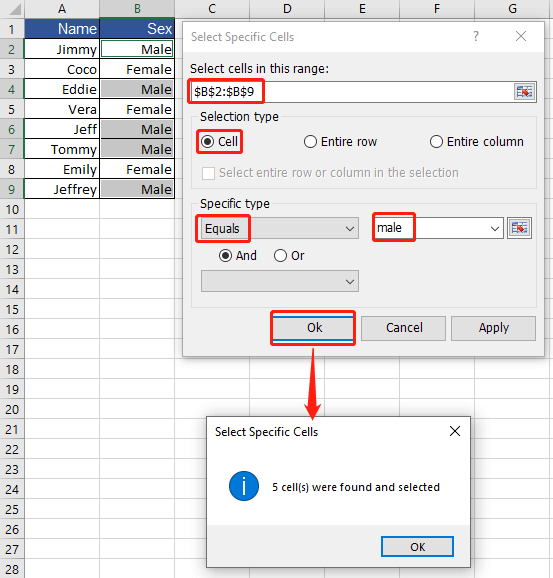The program will return the count result and select the cell(s) that meet(s) your criteria. You can copy them if you need.

• To count the number of names that contain the characters “jeff”, click Contains on the drop-down list, and type jeff, or you can select the cell A6 in the worksheet by clickingbutton.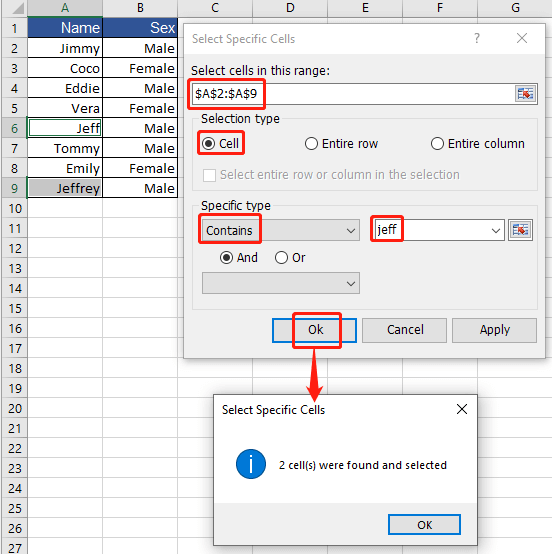The program will return the count result and select the cell(s) that meet(s) your criteria. You can copy them if you need.

## The Best Office Productivity Tools

### Kutools for Excel Solves Most of Your Problems, and Increases Your Productivity by 80%

• Super Formula Bar (easily edit multiple lines of text and formula); Reading Layout (easily read and edit large numbers of cells); Paste to Filtered Range...
• Merge Cells/Rows/Columns and Keeping Data; Split Cells Content; Combine Duplicate Rows and Sum/Average... Prevent Duplicate Cells; Compare Ranges...
• Select Duplicate or Unique Rows; Select Blank Rows (all cells are empty); Super Find and Fuzzy Find in Many Workbooks; Random Select...
• Exact Copy Multiple Cells without changing formula reference; Auto Create References to Multiple Sheets; Insert Bullets, Check Boxes and more...
• Favorite and Quickly Insert Formulas, Ranges, Charts and Pictures; Encrypt Cells with password; Create Mailing List and send emails...
• Extract Text, Add Text, Remove by Position, Remove Space; Create and Print Paging Subtotals; Convert Between Cells Content and Comments...
• Super Filter (save and apply filter schemes to other sheets); Advanced Sort by month/week/day, frequency and more; Special Filter by bold, italic...
• Combine Workbooks and WorkSheets; Merge Tables based on key columns; Split Data into Multiple Sheets; Batch Convert xls, xlsx and PDF...
• Pivot Table Grouping by week number, day of week and more... Show Unlocked, Locked Cells by different colors; Highlight Cells That Have Formula/Name...### Office Tab - brings tabbed interface to Office, and make your work much easier

• Enable tabbed editing and reading in Word, Excel, PowerPoint, Publisher, Access, Visio and Project.
• Open and create multiple documents in new tabs of the same window, rather than in new windows.
• Increases your productivity by 50%, and reduces hundreds of mouse clicks for you every day!